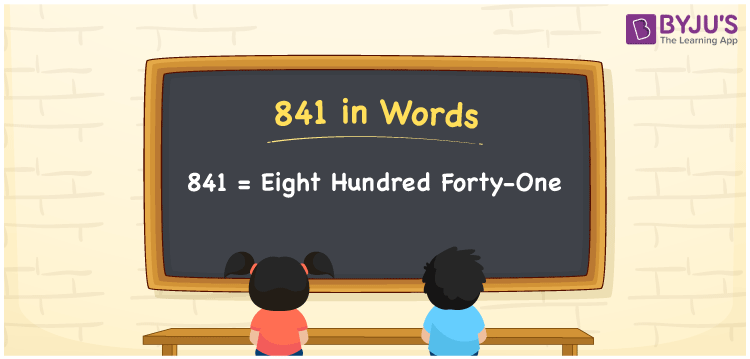# 841 in Words

841 in words is “Eight hundred forty-one”. The natural number 841 has three-digits and it lies between 840 and 842. To transform the number into words, we generally prefer the place value system. In this article, let us have a look at the procedure of writing the number 841 in words using the place value system in detail.

 841 in Words: Eight Hundred Forty-one. Eight Hundred Forty-one in Numerical Form: 841.

## 841 in English Words## How to Write 841 in Words?

Go through the below table to learn the place values of 841.

 Hundreds Tens Ones 8 4 1

The expanded form of 841 is as follows:

= 8 × Hundred + 4 × Ten + 1 × One

= 8 × 100 + 4 × 10 + 1 × 1

= 800 + 40 + 1

= 841

= Eight hundred forty-one

Hence, 841 in words is eight hundred forty-one.

841 in words – Eight hundred forty-one

Is 841 an odd number? – Yes

Is 841 an even number? – No

Is 841 a perfect square number? – Yes

Is 841 a perfect cube number? – No

Is 841 a prime number? – No

Is 841 a composite number? – Yes

## Frequently Asked Questions on 841 in Words

Q1

### How to spell 841 in words?

841 in words is eight hundred forty-one.

Q2

### Simplify 800 + 41, and express it in words.

Simplifying 800 + 41, we get 841. Hence, 841 in words is eight hundred forty-one.

Q3

### Is 841 a perfect square number?

Yes, 841 is a perfect square number.